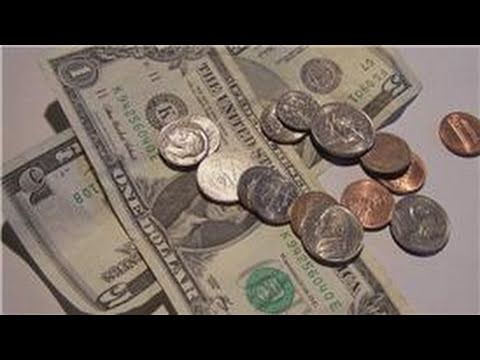# Math Tricks You Could Use in Your Daily Life

Share via

Many of us–even engineers–still struggle with math on a daily basis. Some struggle with calculating fractions in their heads, whiles some have trouble computing all the money they make for a living. Well, here are some math tricks you could use for your daily life.

Find the Annual Cost of Your CoffeeSource: Officecoffeepros
Coffee lovers find that coffee is always part of their budget, but knowing the exact amount of money you spend on it per year can be challenging. This trick allows you get a rough estimate of how much you spend on this elixir every year.

Say each cup of coffee costs \$4 and you drink it 5 times a week, that’s already \$20 out of your pocket. Now take that \$20, add two zeros in the end and you have \$2000. Now divide that by two and you have an estimated cost of coffee per year! Now that’s an expensive routine.

Calculate a TipAny good American would urge you to leave a tip at a restaurant after a good meal. These tips range anywhere from 10-25 percent of the total cost. If you want to be able to get this number right without a calculator, this math trick is for you.
Let’s say your bill is \$25, then 15% of that would be like:

(\$25 * 0.10) + ((\$25 * 0.10) / 2) = 2.5 + 1.25 = 3.75

That looks really daunting, but this trick can pull you through, take the amount and the percentage then move the decimal place once to the left. Multiply both and voila!

2.5 x 1.5 = 3.75

Read more  What This Study of 1,000 CEOs Means for Engineering Managers

Dividing Numbers By 5Source: Puzderko

Dividing by 5 for small numbers is very simple, it’s the larger numbers that prove more difficult. Take the number 215 for example, it’s not that simple to do manually. But I assure you, with this trick, you’ll be able to get the answer really fast.

All you have to do is take the number, multiply it by 2, then move the decimal once to the left
215 x 2 = 430 → 43

It’s really easy. If you have a number that’s not evenly divisible by 5, it can still apply and give you a good answer up to a single decimal point. (e.g. 423 x 2 = 846 → 84.6)

Turn Repeating Decimals Into Fractions

Getting the fraction form of a repeating decimal like 0.63636363 can be hard. But if you take the repeating part, 63, then divide it by the same amount of decimal places of 9s, you’ll be able to see it much clearer.
63/99 = 7/11

This trick will most likely not be useful without a regular calculator, but it’s really handy to use if you don’t have a scientific one on hand. You have to agree that 7/11 is way easier to read than 0.63636363636363636363636363…, right?

Convert Between Fahrenheit and CelsiusSource: Mountain Safety

If you’re the type who likes to travel to different places, it’s good to know how to convert from Celsius to Fahrenheit since different countries use either as their temperature standards. This can really be annoying for travelers, so here’s how to convert between temperatures really quick. It’s not an exact conversion but, it will give you a fairly accurate temperature.

For C to F, multiply the temperature by two and then add 30 to it

For F to C, subtract 30 and divide by 2

If you’re the math wizard type who wants exact values, just replace 2 by 1.8 and 30 by 32 and repeat the process.

Share via

Read more  Stephen Hawking: Revisiting The Life of One of Science’s Greatest